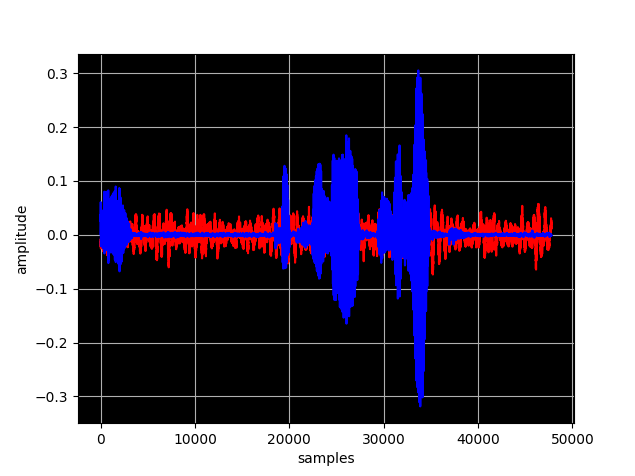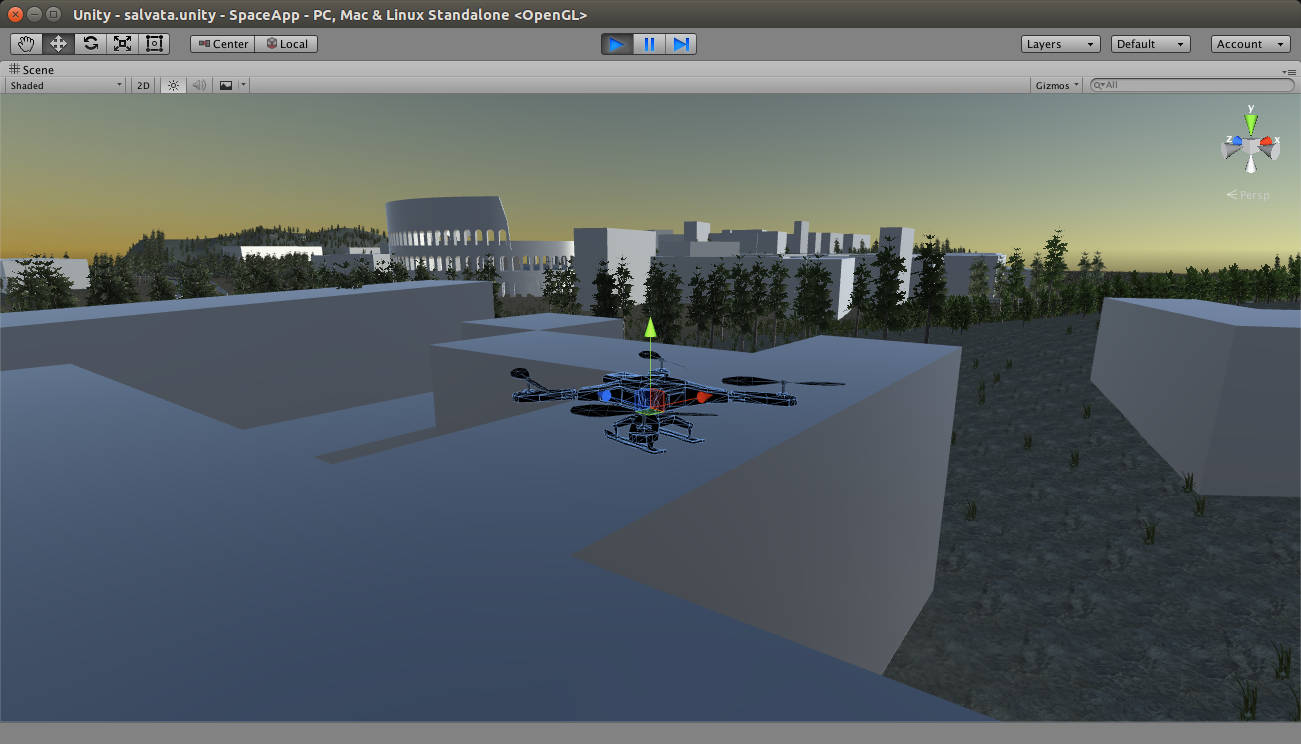# python

•## Manipulating audio files using Python and Linux

Tag: , , , , , , , |

In this post we are going to manipulate some wav files using Python. We would like to add some noise or sound to a recorded wav file. Record your voice Let's record a sound sample of your voice using the rec command userk@dopamine:~$mkdir temp && cd temp userk@dopamine:~/temp$ rec voice.wav Input File :

•## Hotword spotting with SnowBoy Kitt.ai Part I

Snowboy is a hotword detection engine from Kitt ai. They have prepared a easy to use vocal command recogniton that can be used straight in you project. If you have a company or a start up contact them. What we want to do This post is the first part of the guide. We will

•## Python – Scikits Candidate Optimal trajectory

|

On the last article we have installed the Python Scikits library and run the samples provided. In this quick article we would like to find a candidate optimal trajectory that minimizes a weighted combination of speed and position values during a considered time interval $t_0=0$ s and $t_f=10$s. The performance measure $J(u)$ looks like

•## Python – Scikits Two Point Boundary Value Problem

|

An application of Calculus of Variation is the determination of control action $u(t)$ such that a system described by a differential equation system $$\dot{x}(t) = {a(x(t),u(t),t)}.$$ follows a trajectory $x(t)$ for all time instants that minimizes a performance measure $J(u)$ defined by $$J(u) = \int_{t0}^{tf} {g(x(t),u(t),t)} dt$$ A wonderful application could be a tridimentional trajectory